# nowhere-zero flow

## The intersection of two perfect matchings ★★

Author(s): Macajova; Skoviera

Conjecture   Every bridgeless cubic graph has two perfect matchings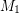,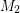so thatdoes not contain an odd edge-cut.

Keywords: cubic; nowhere-zero flow; perfect matching

## Half-integral flow polynomial values ★★

Author(s): Mohar

Let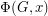be the flow polynomial of a graph. So for every positive integer, the value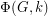equals the number of nowhere-zero-flows in.

Conjecturefor every 2-edge-connected graph.

Keywords: nowhere-zero flow

## A nowhere-zero point in a linear mapping ★★★

Author(s): Jaeger

Conjecture   Ifis a finite field with at least 4 elements andis an invertible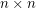matrix with entries in, then there are column vectorswhich have no coordinates equal to zero such that.

Keywords: invertible; nowhere-zero flow

## Unit vector flows ★★

Author(s): Jain

Conjecture   For every graphwithout a bridge, there is a flow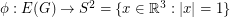.

Conjecture   There exists a map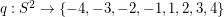so that antipodal points ofreceive opposite values, and so that any three points which are equidistant on a great circle have values which sum to zero.

Keywords: nowhere-zero flow

## Real roots of the flow polynomial ★★

Author(s): Welsh

Conjecture   All real roots of nonzero flow polynomials are at most 4.

Keywords: flow polynomial; nowhere-zero flow

## A homomorphism problem for flows ★★

Author(s): DeVos

Conjecture   Letbe abelian groups and let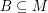andsatisfyand. If there is a homomorphism from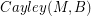to, then every graph with a B-flow has a B'-flow.

Keywords: homomorphism; nowhere-zero flow; tension

## The three 4-flows conjecture ★★

Author(s): DeVos

Conjecture   For every graphwith no bridge, there exist three disjoint sets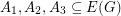withso that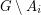has a nowhere-zero 4-flow for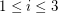.

Keywords: nowhere-zero flow

## Bouchet's 6-flow conjecture ★★★

Author(s): Bouchet

Conjecture   Every bidirected graph with a nowhere-zero-flow for some, has a nowhere-zero-flow.

Keywords: bidirected graph; nowhere-zero flow

## Jaeger's modular orientation conjecture ★★★

Author(s): Jaeger

Conjecture   Every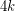-edge-connected graph can be oriented so that(mod) for every vertex.

Keywords: nowhere-zero flow; orientation

## 3-flow conjecture ★★★

Author(s): Tutte

Conjecture   Every 4-edge-connected graph has a nowhere-zero 3-flow.

Keywords: nowhere-zero flow